Open In App

# Spatial Aptitude

Spatial aptitude refers to the ability to think and draw conclusions about objects in 3-Dimensional space. Aptitude skills like this enhance reasoning which can help to imagine and differentiate between real-world objects in the head. Thus, spatial aptitude is used to test spatial intelligence.

### Translation:

It means how much a point is moved to the left or right and up or down. Translation of coordinates based on certain rules resulting in a new image is what is asked mostly in these types of questions.

Example: In a coordinate plane, triangle A(3,-1) B(7,-5) C(-2,-2) has shifted 4 units to the left and 5 units up. Determine the coordinates of triangle A’B’C’.

Solution: As the triangle, ABC has shifted 4 units to the left and 5 units up which means we need to subtract 4 units from the x-axis and add units. This is our translation rule for the above problem. (x,y) ⇢(x-4,y+5)

Thus, the coordinates of A’B’C’ are: A'(-1,4) B'(3,0) C'(-6,3).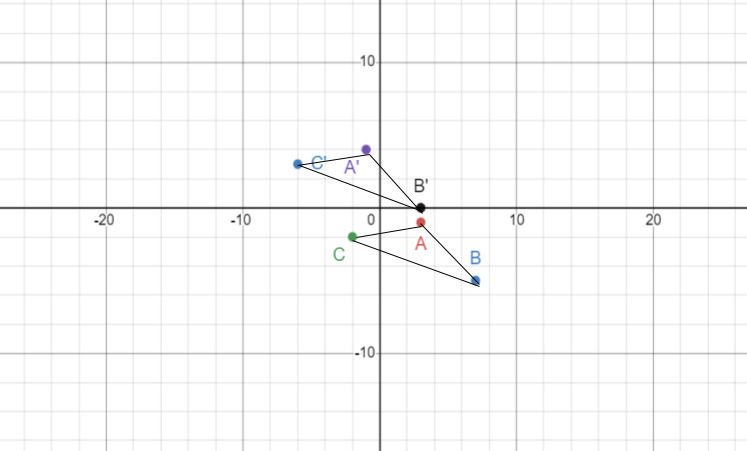Translation

### Rotation:

It means how the object would look when rotated. It requires the ability of mental rotation.

Example: If we rotate the topmost figure clockwise by 90 degrees once we get the figure in option A and if we rotate it clockwise by 90 degrees twice then we get the figure in option D. Thus, the solution is option A, and D.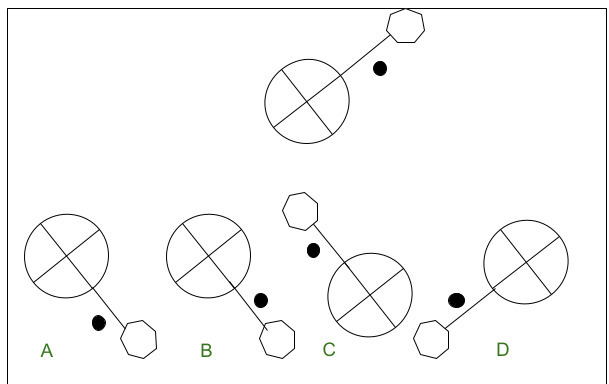Rotation

### Mirroring:

It means how the object would look when inverted about the mirror line. This concept focuses on the ability to visualize laterally inverted objects.

Example: How will the word TRIANGLE look when reflected on the Y-axis?

If we look closely, after reflection on the Y-axis the right side of the letters appears as the left side and vice-versa. Therefore, the third option is the correct image after reflection.

Solution: Ǝ⅃ӘИAIЯT

### Assembling

Assembling means assembling different shapes/objects together based on certain rules to make another shape/object.

Example: When we assemble the three different shapes by connecting the dots in proper order we find it to look like the assembled object in Solution: The straight-line ab connects the center of the semi-circle-like object with the corner point of the triangle.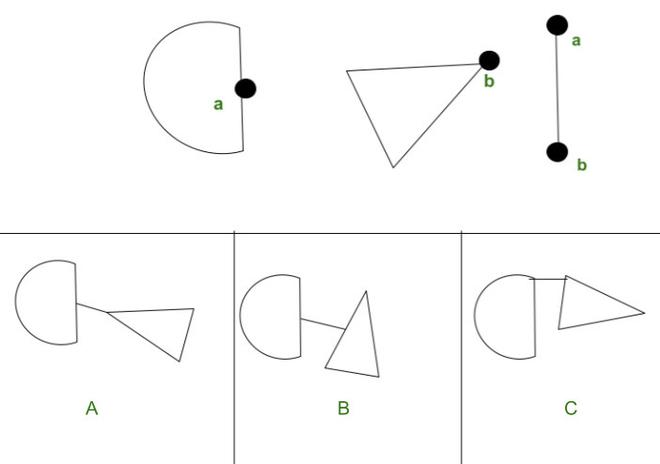Assembling

### Grouping:

It means categorizing objects based on identical properties. The properties can be related to angle, no of sides, shapes, etc.

Example: 1,5 and 7 form one group because all of them have 3 sides. 2,4, and 9 form another group because they all have 4 sides. Similarly, 3,6, and 8 form a group as they have a circle as a base.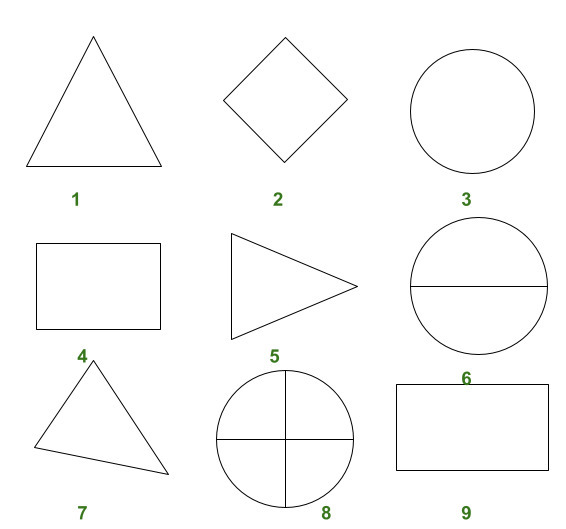Grouping

### Paper folding and cutting:

It means how a piece of paper may look after performing certain cut and fold operations on it. The dotted lines in these problems indicate the reference line along which the paper is folded and the arrow indicates the direction of the fold/unfold.

Example: In the leftmost image, when the paper is unfolded about the dotted lines, the punched holes appear in the following manner: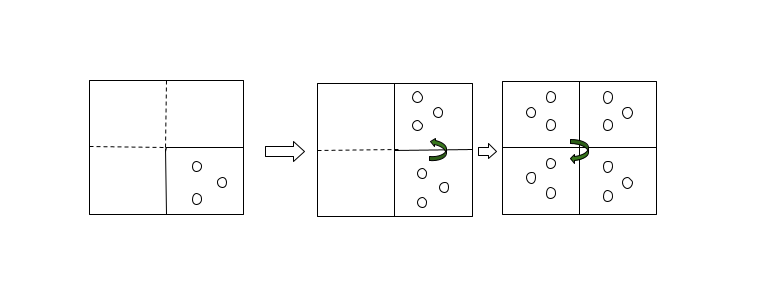Paper Folding

### Patterns in 2 and 3 dimensions:

It means deriving logical conclusions from a given pattern to find a missing pattern in the sequence.

Example: If we observe the pattern in which the top 5 objects appear, we will find that 1 or 2 triangles forming the star are erased alternately in a clockwise manner. Therefore the 6th object in the sequence will be option B.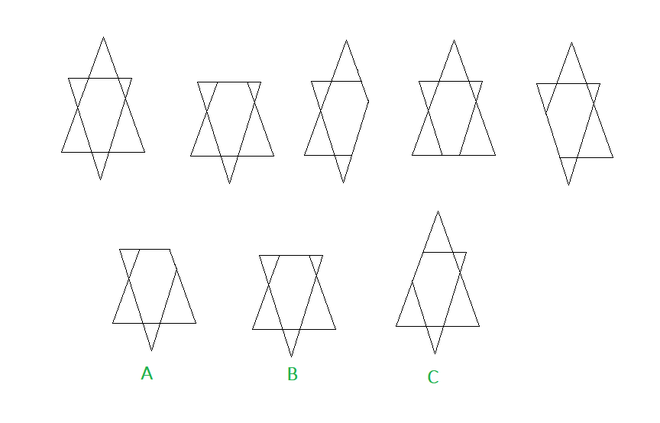Patterns